# Direct proof of a theorem about compact funcoids

 Importance: Medium ✭✭
 Author(s): Porton, Victor
 Subject: Topology
 Keywords: compact space compact topology funcoid reloid uniform space uniformity
 Recomm. for undergrads: no
 Posted by: porton on: February 8th, 2014
Conjecture   Letis a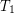-separable (the same as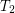for symmetric transitive) compact funcoid and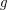is a uniform space (reflexive, symmetric, and transitive endoreloid) such that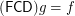. Then.

The main purpose here is to find a direct proof of this conjecture. It seems that this conjecture can be derived from the well known theorem about existence of exactly one uniformity on a compact set. But that would be what I call an indirect proof, we need a direct proof instead.

The direct proof may be constructed by correcting all errors an omissions in this draft article.

Direct proof could be better because with it we would get a little more general statement like this:

Conjecture   Letbe a-separable compact reflexive symmetric funcoid andbe a reloid such that
\item; \item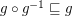.

Then.

## Bibliography

Victor Porton. Compact funcoids

* indicates original appearance(s) of problem.